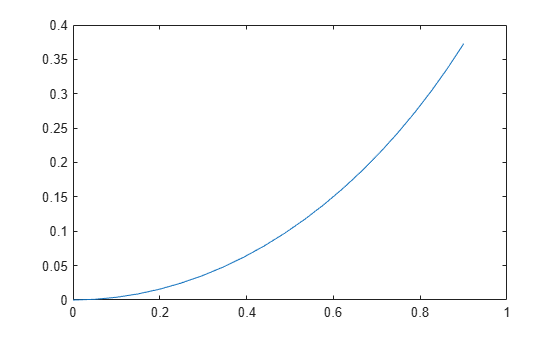Documentation

unicycleKinematics

Unicycle vehicle model

Description

unicycleKinematics creates a unicycle vehicle model to simulate simplified car-like vehicle dynamics. The state of the vehicle is defined as a three-element vector, [x y theta], with a global xy-position, specified in meters, and a vehicle heading angle, theta, specified in radians. This model approximates a unicycle vehicle with a given wheel radius, WheelRadius, that can spin in place according to a heading angle, theta. To compute the time derivative states for the model, use the derivative function with input commands and the current robot state.Creation

Description

example

kinematicModel = unicycleKinematics creates a unicycle kinematic model object with default property values.

kinematicModel = unicycleKinematics(Name,Value) sets additional properties to the specified values. You can specify multiple properties in any order.

Properties

expand all

The wheel radius of the vehicle, specified in meters.

The vehicle speed range is a two-element vector that provides the minimum and maximum vehicle speeds, [MinSpeed MaxSpeed], specified in meters per second.

The VehicleInputs property specifies the format of the model input commands when using the derivative function. Options are specified as one of the following strings:

• "WheelSpeedHeadingRate" — Wheel speed and heading angular velocity, specified in radians per second.

• "VehicleSpeedHeadingRate" — Vehicle speed and heading angular velocity, specified in radians per second.

Object Functions

 derivative Time derivative of vehicle state

Examples

collapse all

Create a Robot

Define a robot and set the initial starting position and orientation.

kinematicModel = unicycleKinematics;
initialState = [0 0 0];

Simulate Robot Motion

Set the timespan of the simulation to 1 s with 0.05 s timesteps and the input commands to 10 m/s and left turn. Simulate the motion of the robot by using the ode45 solver on the derivative function.

tspan = 0:0.05:1;
inputs = [10 1]; %Constant speed and turning left
[t,y] = ode45(@(t,y)derivative(kinematicModel,y,inputs),tspan,initialState);

Plot path

figure
plot(y(:,1),y(:,2))Lynch, Kevin M., and Frank C. Park. Modern Robotics: Mechanics, Planning, and Control 1st ed. Cambridge, MA: Cambridge University Press, 2017.

Robotics System Toolbox DocumentationGet trial now